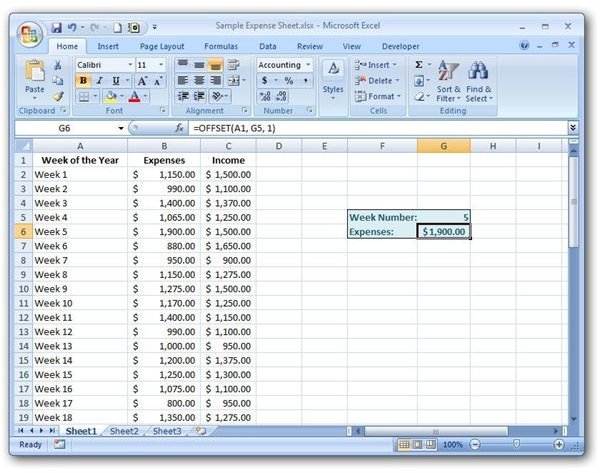# How to Use the OFFSET Function in Microsoft Excel

Page content

## Basics of the OFFSET Function

The OFFSET function is a special type of lookup function in Excel that allows users to find a value in a table based on a particular reference point. In theory, this tool is similar to the LOOKUP function in that it can be used to retrieve information about a specific entry in a table. However, instead of stating a result vector to use for evaluation of the function, the OFFSET function specifies how far to move from the initial reference point (in terms of columns and rows) in order to find the function value.

Syntax of the OFFSET Function

The basic syntax of the OFFSET function is

OFFSET(reference, rows, cols, height, width)

where the arguments of the function of the following meanings.

• reference – The cell or group of cells that you want to use as the initial reference point for the OFFSET function. Though you can pick any reference point you like, it’s common to use one of the “corner” cells of the table or list that you are querying.

• rows – This is a numeric value that represents the number of rows you want to move to find your OFFSET function value. If you are moving down one or more rows, this value should be positive. If moving up, then the value will be negative.

• cols – Like the rows argument, this is a numeric value that represents the number of columns you want to move to find the function result. If moving to the right, this value should be positive. Negative values represent moving to the left.

• height – This value is optional and denotes the number of rows you want included in the OFFSET function result. If no value is given, it will default to 1.

• width – This value is optional as well and denotes the number of columns you want to include in the OFFSET function result. When no value is specified, it will default to 1.

Now, let’s look at an example to explain what this really means.

How to Use the OFFSET Function

For our example, we’ll use a table that contains information on the amount of expenses paid out and income received on a weekly basis. A screenshot of this information is shown below. (Click any image for a larger view.)

We would like to create an OFFSET function that can take a week number that is entered by the spreadsheet user and return the expenses paid out during that same time period.

As the initial reference value, we will use the upper left-hand corner of the table shown in the screenshot above, or cell A1. We will ask the spreadsheet user to enter the week number in cell G5, so this value will represent the rows argument as it is the number of rows down we want to move from cell A1. If you move one column to the right, you will find the expense information. Thus, our cols value will be 1. Since we only want that a single cell (height 1 and width 1) returned, we don’t have to enter any information for the height and width arguments.

This OFFSET function can be stated as:

OFFSET(A1, G5, 1)

The screenshot below shows how this would be entered into Excel.